# 1. 什么是Matplotlib

Matplotlib 是一个 Python 的 2D 绘图库，通过 Matplotlib ，开发者可以仅需要几行代码，便可以生成折线图，直方图，条形图，饼状图，散点图等。

# 2. 安装和基本使用

``````import matplotlib.pyplot as plt
import numpy as np

y1 = [np.random.randint(0,10) for x in range(20)]
x1 = range(5,25)
plt.plot(x1,y1)
``````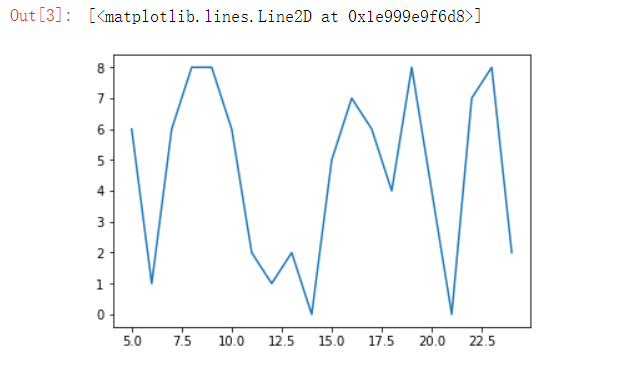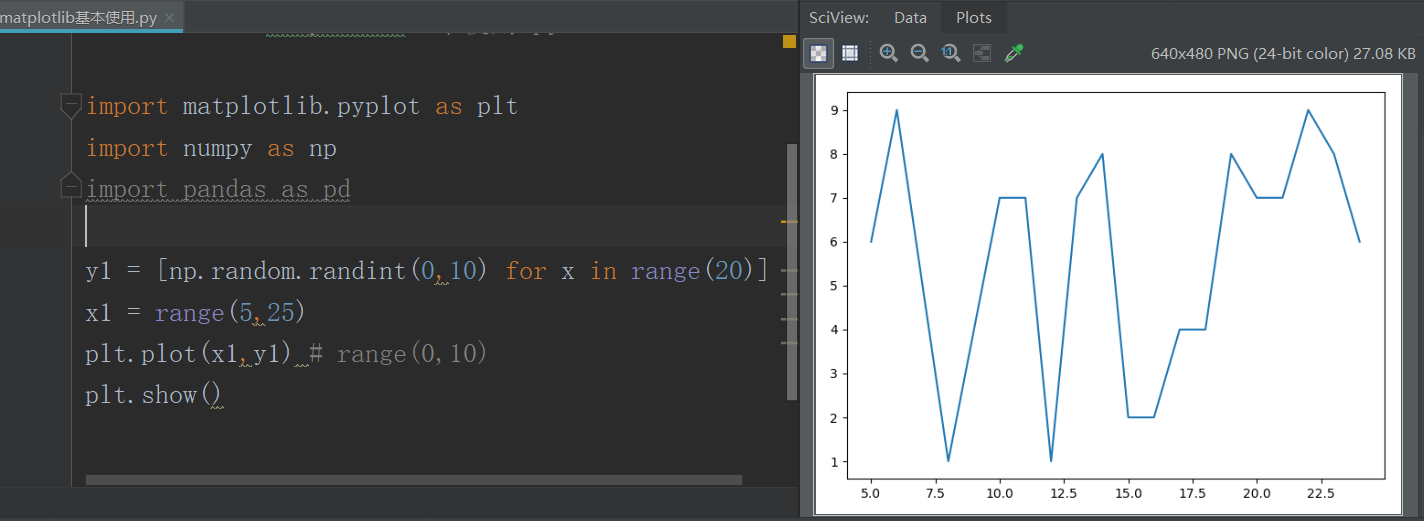# 3.点线缩写和颜色的选择

``````import matplotlib.pyplot as plt
import numpy as np

y1 = [np.random.randint(0,10) for x in range(20)]
x1 = range(5,25)
plt.plot(x1,y1)
``````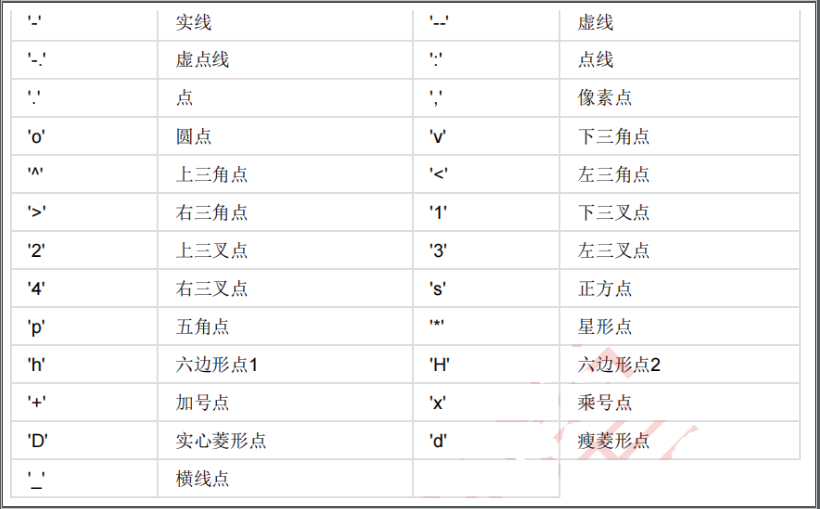[En]

In addition to setting the shape of the line, we can also set the color of the point. In general, there are three ways to set the color of the line:

1. 第一种是使用颜色名称（ r 是 red 的缩写）的形式；
2. 第二种是使用十六进制的方式；
3. 第三种是使用 RGB 或 RGBA 的方式。

``````plt.plot([1,2,3,4,5],[1,2,3,4,5],'r') #&#x5C06;&#x989C;&#x8272;&#x7EBF;&#x6761;&#x8BBE;&#x7F6E;&#x6210;&#x7EA2;&#x8272;
plt.plot([1,2,3,4,5],[1,2,3,4,5],color='red') #&#x5C06;&#x989C;&#x8272;&#x8BBE;&#x7F6E;&#x6210;&#x7EA2;&#x8272;

plt.plot([1,2,3,4,5],[1,2,3,4,5],color='#000000') #&#x5C06;&#x989C;&#x8272;&#x8BBE;&#x7F6E;&#x6210;&#x7EAF;&#x9ED1;&#x8272;
plt.plot([1,2,3,4,5],[1,2,3,4,5],color=(0,0,0,0)) #&#x5C06;&#x989C;&#x8272;&#x8BBE;&#x7F6E;&#x6210;&#x7EAF;&#x9ED1;&#x8272;
``````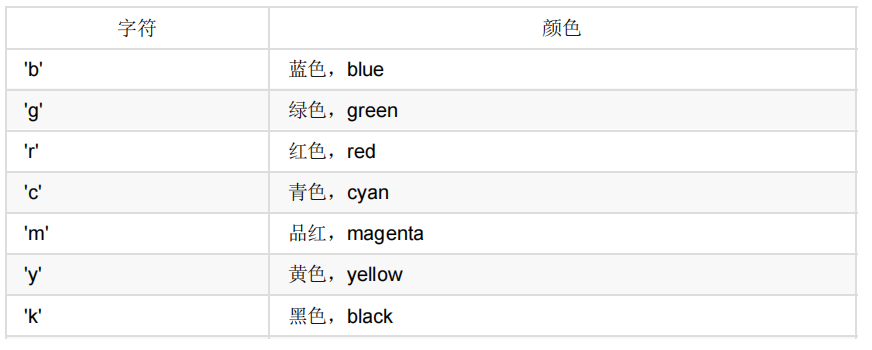[En]

If you are using a color name, you can write it in the same string as the shape of the line. For example, if you use red five-corner points, you can do it in the following ways: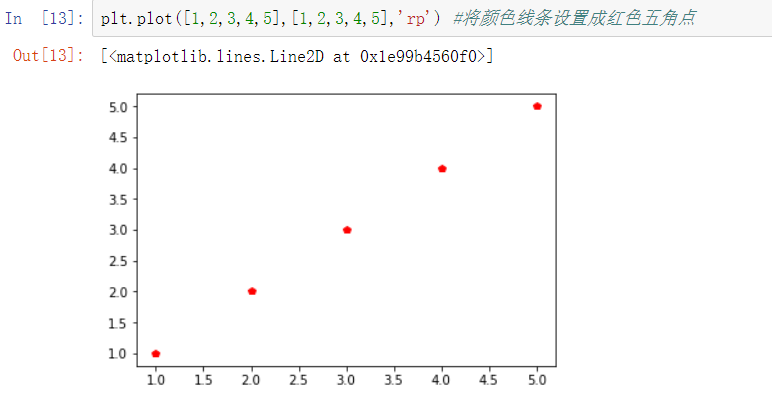[En]

So if I want to change the line of the original picture to a red dotted line, then I can do it through the following code:

``````y1 = [np.random.randint(0,10) for x in range(20)]
x1 = range(5,25)
plt.plot(x1,y1,"--",color='r')
``````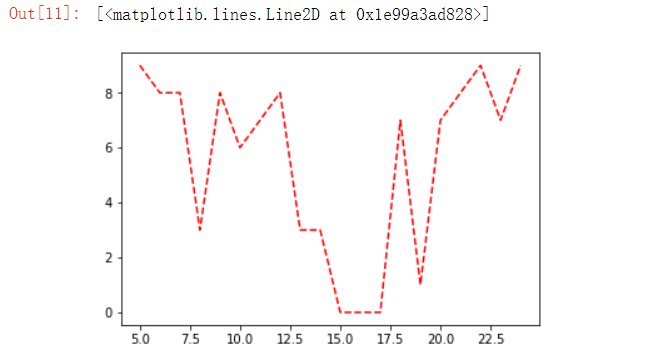1. plt.plot可以只传Y轴的值，如果只传Y轴的值，那么X轴就会默认使用range(0,Y的长度)
2. plt.plot的x和y参数不能够作为关键字参数来传递，只能作为位置参数来传。
3. plt.plot中的data参数可以为一个字典或者DataFrame对象，然后在x和y上指定这个列的名字，那么plot会自动读取。这里有一个细节，因为x,y,fmt都是在前面，所以如果只传x和y，那么可能会产生歧义，这时候我们可以多传一个空的参数作为fmt的参数，就不会有警告了。
4. plt.plot的fmt参数可以设置线条的样式以及颜色。
5. plt.plot的color参数可以使用字母、十六进制，或者是RGBA的方式来设置颜色。
6. plt.plot函数的官方文档

# 4. 设置图的信息

## 4.1 设置线条样式的方法

1. 使用 plot 方法：

plot 方法就是用来绘制线条的，因此可以在绘制的时候就把线条相关的样式通过参数传进去。

``````y = [np.random.randint(0,10) for x in range(10)]
plt.plot(y,lw=4,c='r',ls='--',aa=True)
``````

c就是颜色color的首字母，r就是红色red
ls即linestyle，表示点线格式，我们这里用虚线展示。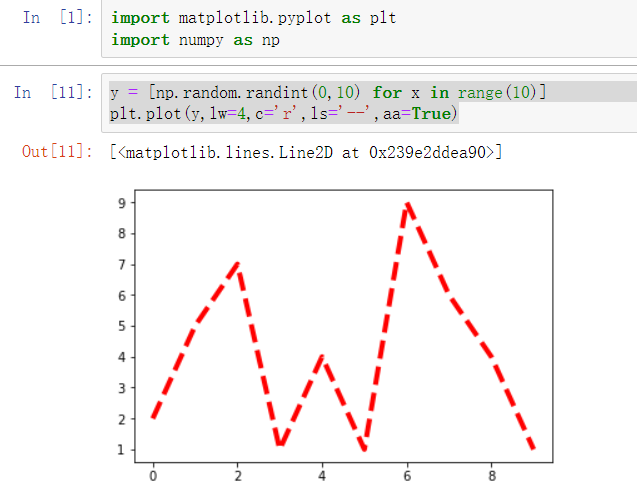1. 通过 Line2D 对象来设置：

plot 方法会返回一个装有 Line2D 对象的列表，比如 lines=plt.plot(x1,y1,x2,y2) 因为绘制了两根线条，因此 lines 中会有两个 2D 对象。而如果 plot 只绘制一根线条，那么 lines 中就只有一个 Line2D 对象。拿到这个 Line2D 对象后就可以通过 set_属性名 设置线条的样式了

``````y1 = [np.random.randint(0,10) for x in range(10)]
y2 = [np.random.randint(0,10) for x in range(10)]
lines = plt.plot(range(10),y1,range(10),y2)
line = lines
line.set_color('r')
line.set_linewidth(4)
line.set_alpha(0.5)
``````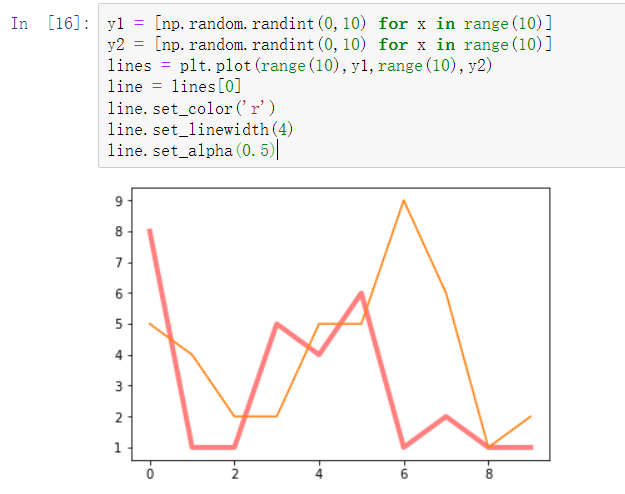3. 使用 plt.setp 来设置： setp 的好处是一次性可以设置多根线条的样式。

``````y1 = [np.random.randint(0,10) for x in range(10)]
y2 = [np.random.randint(0,10) for x in range(10)]
lines = plt.plot(range(10),y1,range(10),y2)
plt.setp(lines,linewidth=4)
``````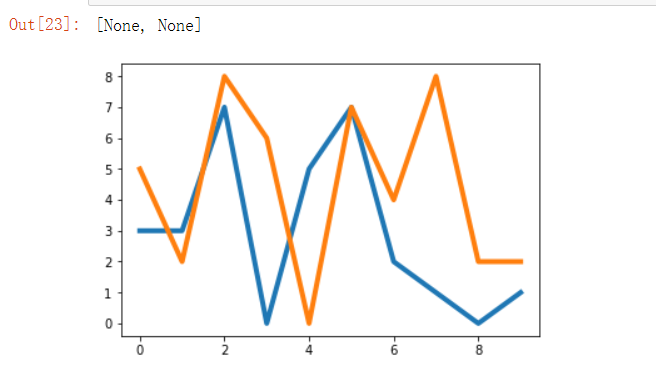1. 使用plot绘图的时候，就可以传递Line2D的属性值进去，来修改线条的样式。
2. 使用plot返回的线条，单独去设置也可以，但是单独设置的时候，是通过set_Line2D属性名()的方式来设置的。
3. 使用plt.setp来一次性设置多个线条的样式。

## 4. 2 设置轴和标题

### 4.2.1 设置轴名称

`````` plt.plot(x,y,linewidth=10,color='red')
plt.xlabel("x&#x8F74;")
plt.ylabel("y&#x8F74;")
``````

``````# &#x52A0;&#x8F7D;&#x5B57;&#x4F53;
font = font_manager.FontProperties(fname="C:\Windows\Fonts\msyh.ttc")
plt.plot(x,y,linewidth=10,color='red')
plt.xlabel("x&#x8F74;",fontproperties=font)
plt.ylabel("y&#x8F74;",fontproperties=font)
``````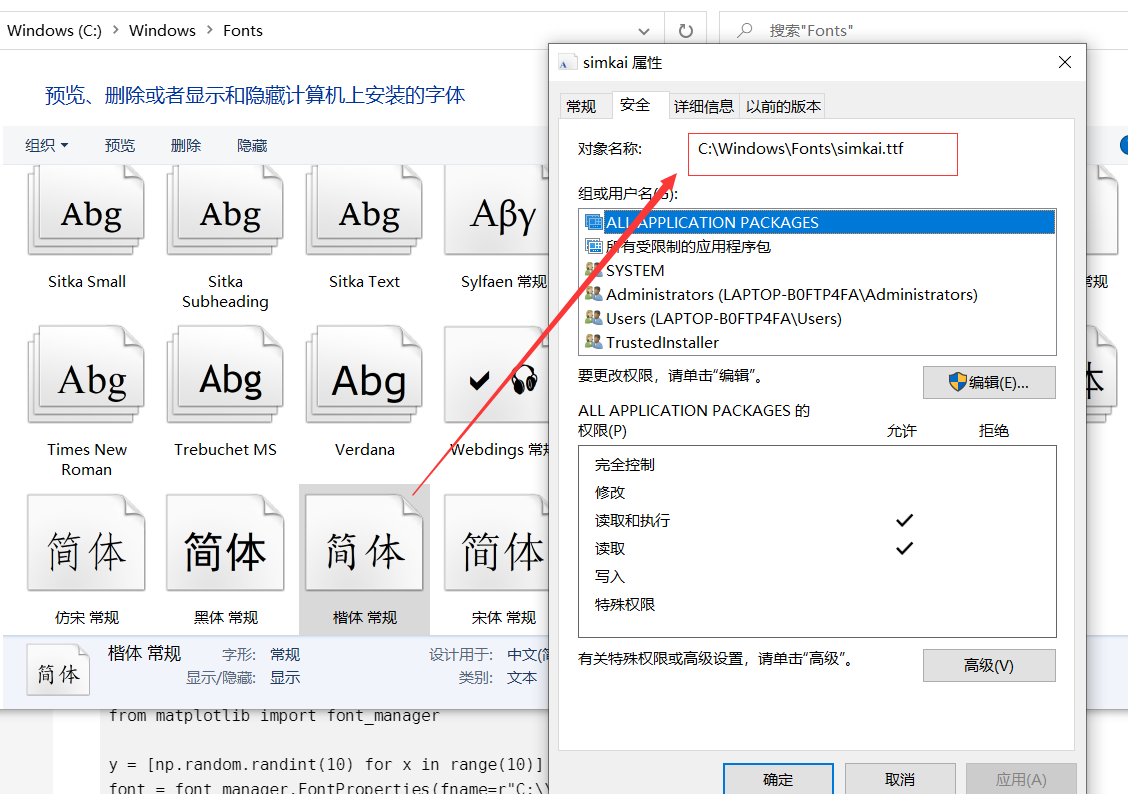### 4.2.2 设置标题

``````import matplotlib.pyplot as plt
import numpy as np
from matplotlib import font_manager

y = [np.random.randint(10) for x in range(10)]
font = font_manager.FontProperties(fname=r"C:\\Windows\\Fonts\\msyh.ttc",size=20)
plt.plot(y)
plt.title("&#x6298;&#x7EBF;&#x56FE;",fontproperties=font)
``````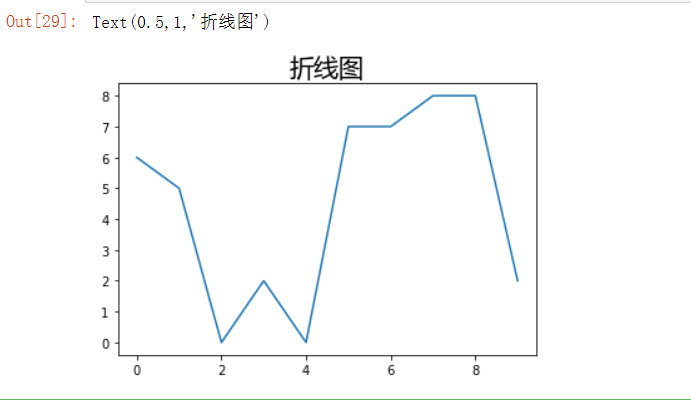### 4.2.3 设置 x 轴和 y 轴的刻度

``````plt.xticks(range(0,20,2)) #&#x5728;x&#x8F74;&#x4E0A;&#x7684;&#x523B;&#x5EA6;&#x662F;0,2,4,6...20
``````

``````_x = range(0,20,2)
_xticks = ["%d&#x5750;&#x6807;"%i for i in _x]
plt.xticks(_x,_xticks,fontproperties=font) #&#x5728;x&#x8F74;&#x4E0A;&#x7684;&#x523B;&#x5EA6;&#x662F;0&#x5750;&#x6807;,2&#x5750;&#x6807;...20&#x5750;&#x6807;
``````

### 4.2.4 复仇者联盟电影票房案例

``````avenger = [17974.4,50918.4,30033.0,40329.1,52330.2,19833.3,11902.0,24322.6,47521.8,32262.0,22841.9,12938.7,4835.1,3118.1,2570.9,2267.9,1902.8,2548.9,5046.6,3600.8]
plt.figure(figsize=(15,5))
plt.plot(avenger,marker="o")
font.set_size(10)
plt.xticks(range(20),["&#x7B2C;%d&#x5929;"%x for x in range(1,21)],fontproperties=font)
plt.xlabel("&#x5929;&#x6570;",fontproperties=font)
plt.ylabel("&#x7968;&#x623F;&#xFF08;&#x5355;&#x4F4D;&#xFF1A;&#x4E07;&#xFF09;",fontproperties=font)
plt.title("&#x590D;&#x4EC7;&#x8005;&#x8054;&#x76DF;&#x524D;20&#x5929;&#x7968;&#x623F;&#x6570;&#x636E;",fontproperties=font)
plt.grid(True)
plt.show()
``````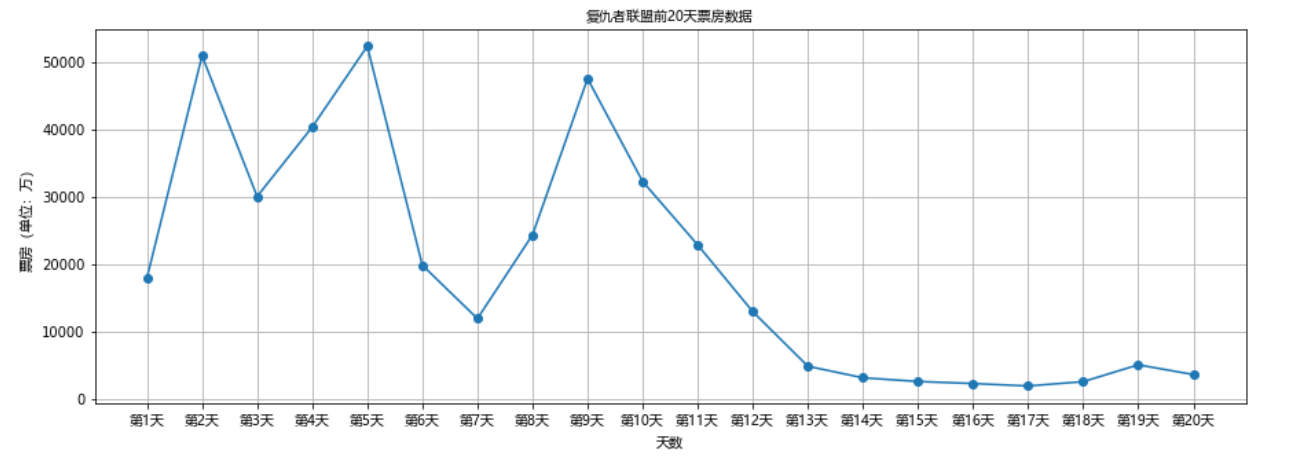### 小结

1. 标题是通过plt.title来设置的。
2. 刻度是通过xticks和yticks来设置的。xticks和yticks需要传递两个参数，第一个参数ticks不重要，只要跟数据量保持一致就可以了，比如有20个数据，那么只要产生20个数据的数组或者列表都可以，第二个参数labels是用来设置每个刻度上的文本显示，这个就比较重了。
3. 轴的名称是通过xlabel和ylabel来设置的。
4. 默认plot是不支持中文的，如果想要支持中文，那么应该创建一个字体对象matplotlib.font_manager.FontProperties，然后指定fname参数，需要具体的路径。这里还有一个小细节，拷贝出来的字体的字符串，前面有一个乱码，要把那个乱码删掉。获取字体路径的方式：右键->属性->安全->对象名称。

# 5. 设置文本注释和marker

## 5.1 设置marker

``````x = np.linspace(0,20)
y = np.sin(x)
plt.plot(x,y,marker="o")
``````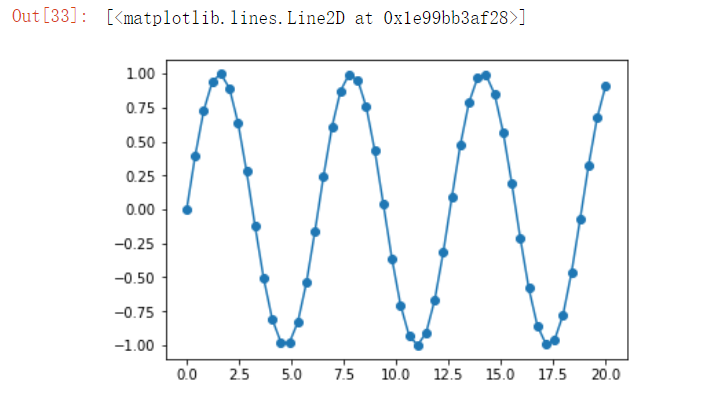``````# &#x4EE5;&#x4E0B;&#x8BBE;&#x7F6E;&#x6807;&#x8BB0;&#x70B9;&#x7684;&#x989C;&#x8272;&#x4E3A;&#x9ED1;&#x8272;&#xFF0C;&#x5C3A;&#x5BF8;&#x4E3A;10
plt.plot(x,y,marker="o",markerfacecolor='k',markersize=10)
``````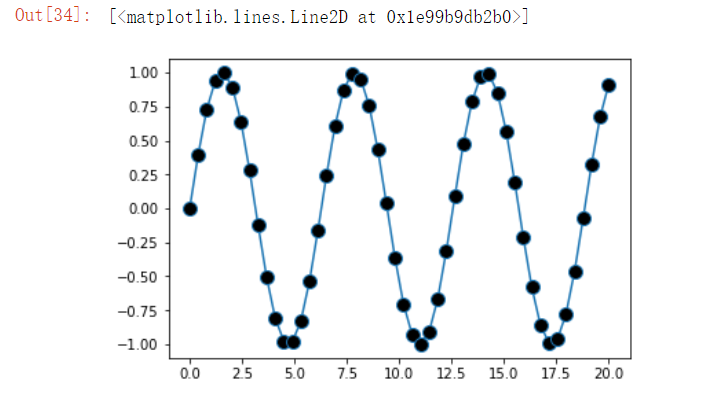## 5.2 设置注释文本

``````y = np.sin(np.arange(20))
plt.plot(y,marker='o')
for index,value in enumerate(y):
plt.annotate("(%d,%.2f)"%(index,value),xy=(index,value),xytext=(index-0.1,value-0.1))
plt.show()
# plt.savefig("./a.png") # &#x4FDD;&#x5B58;&#x56FE;&#x7247;
``````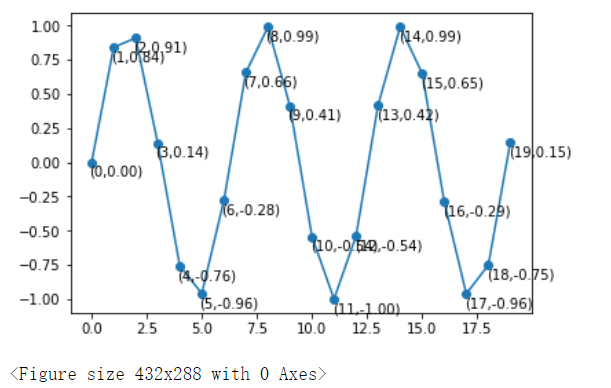## 小结

1. plt.plot的时候，可以通过marker参数设置坐标点的样式。markerfacecolor代表的是点的颜色，markeredgecolor代表的是点的边界的颜色，markersize代表的是点的大小。
2. plt.annotate(text,xy,xytext,arrowprops)是用来做文本注释的，text代表的是需要注释的文本，xy代表的是需要注释的坐标点，xytext代表的是文本的坐标点，arrowprops代表的是箭头的一些属性。
3. annotate的官方文档

# 6. 设置图形样式

## 6.1 调整图片的大小和像素

``````plt.figure(figsize=(10,5),facecolor="r",edgecolor="k",linewidth=2,dpi=200,frameon=True)
plt.plot(np.arange(5,20))
# plt.savefig("./x.png")
``````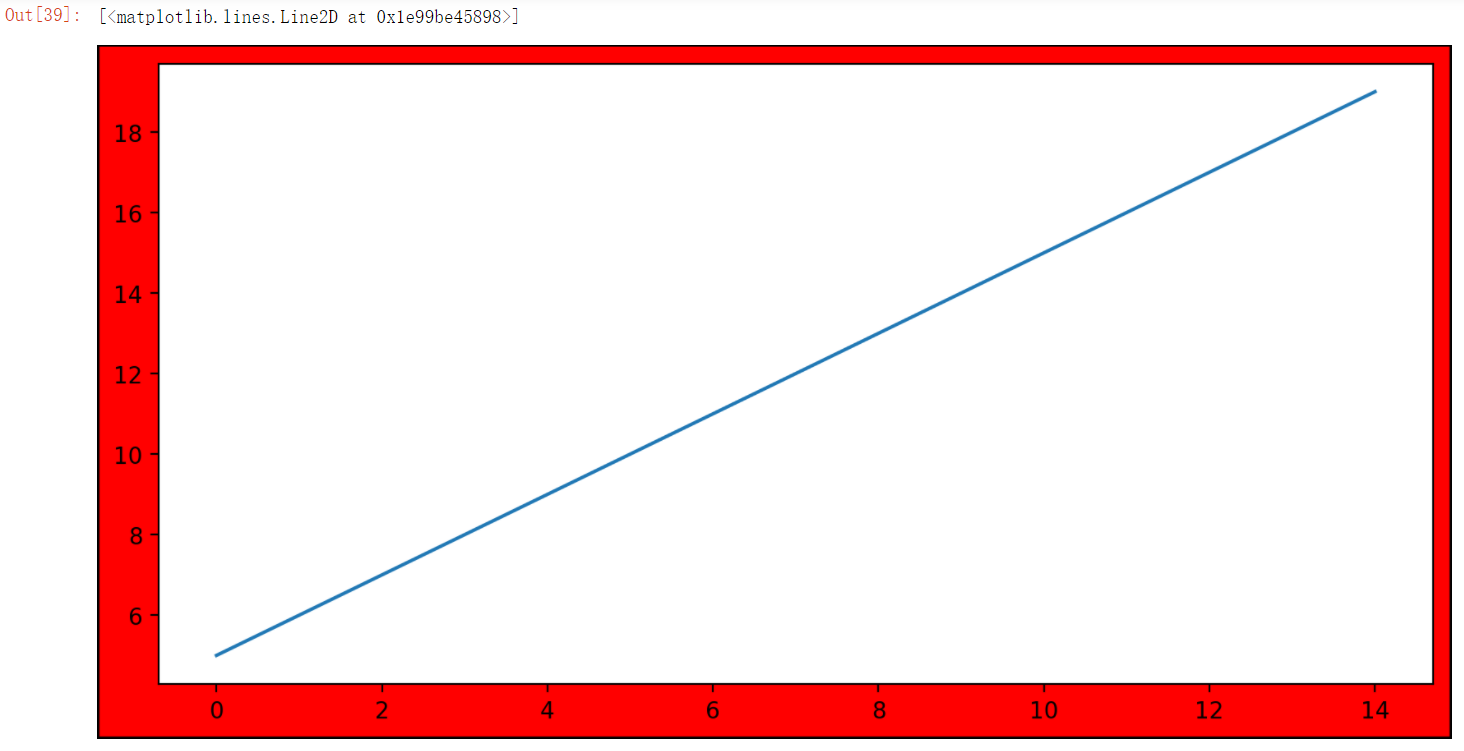## 6.2 显示图片的网格

``````plt.figure(figsize=(10,5),facecolor="r",edgecolor="k",linewidth=2,dpi=200,frameon=True)
plt.plot(np.arange(5,20))
plt.grid()
``````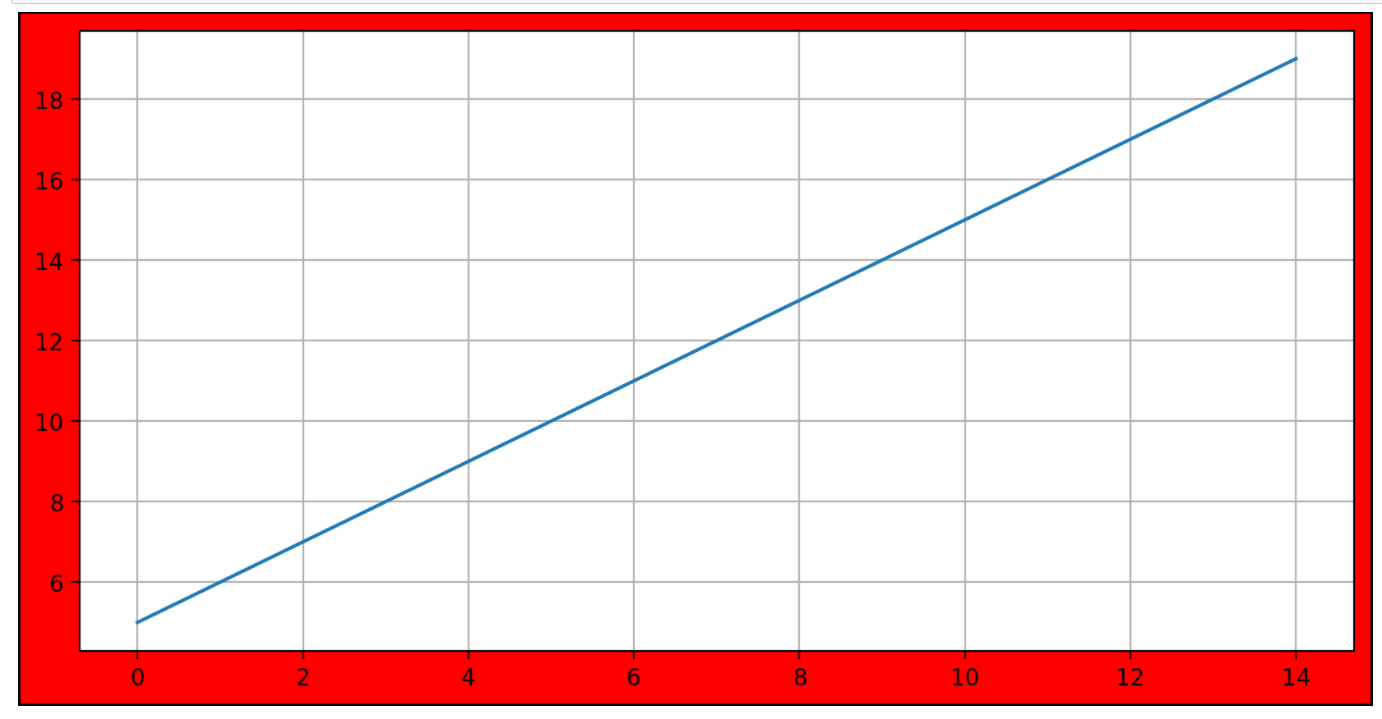## 6.3 绘制多个图

[En]

There are two forms of drawing multiple graphs, the first is to draw multiple lines in one picture, and the second is to draw multiple sub-graphics.

### 6.3.1 绘制多根折线

``````x = np.linspace(0,20)
plt.plot(x,np.sin(x))
plt.plot(x,np.cos(x))
``````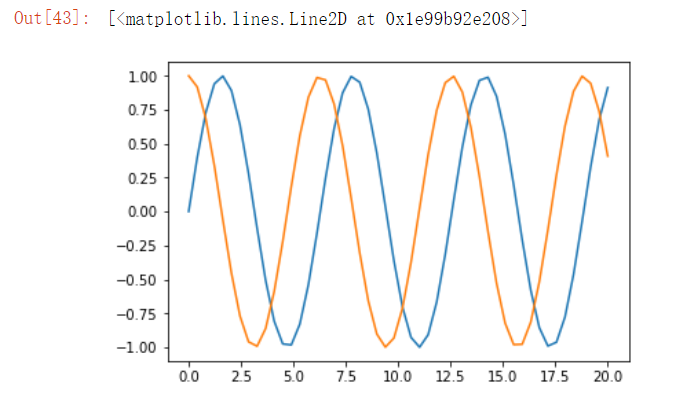### 6.3.2 绘制多个子图

``````plt.subplot(221)
plt.plot(np.arange(10),c='r')
plt.subplot(222)
plt.plot(np.sin(np.arange(10)),c='b')
plt.subplot(223)
plt.plot(np.cos(np.arange(10)),c='y')
plt.subplot(224)
plt.plot(np.tan(np.arange(10)),c='g')
``````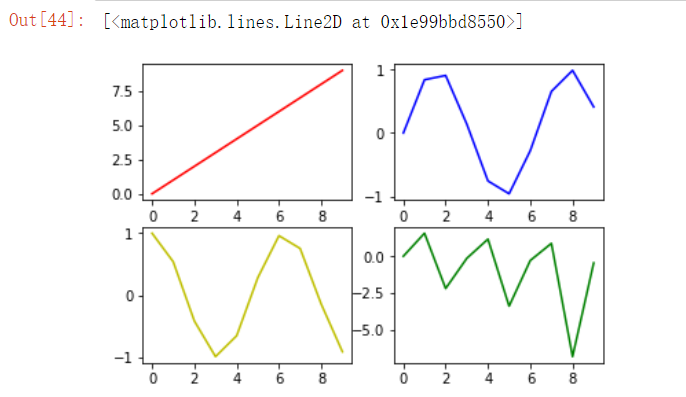``````values = np.linspace(0,20)
plt.style.use("seaborn")
fig,axes = plt.subplots(2,2,sharex=True) #Axes
ax1 = axes[0,0]
ax1.plot(values)
ax2 = axes[0,1]
ax2.plot(np.sin(values),c='y')
ax3 = axes[1,0]
ax3.plot(np.cos(values),c='b')
ax4 = axes[1,1]
ax4.plot(np.tan(values),c='g')
``````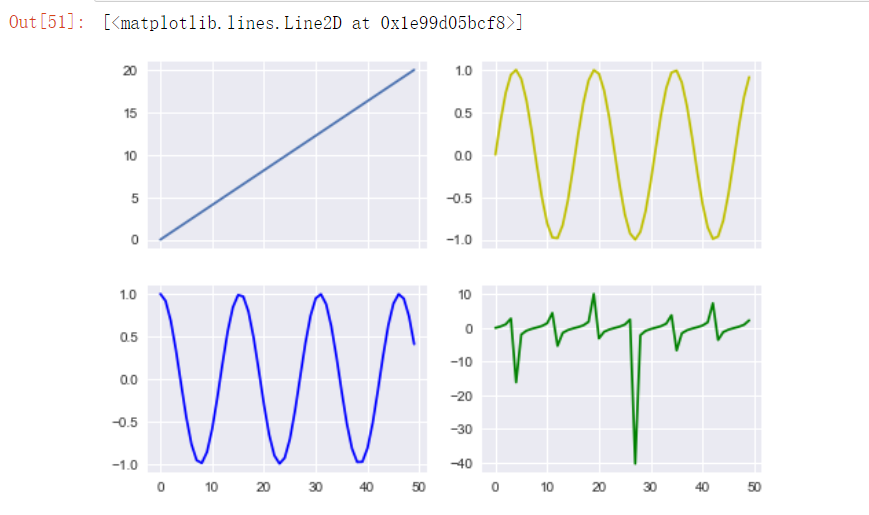``````figure,axes = plt.subplots(2,2,sharex=True,sharey=True)
axes[0,0].plot(np.sin(np.arange(10)),c='r')
axes[0,1].plot(np.cos(np.arange(10)),c='b')
axes[1,0].plot(np.tan(np.arange(10)),c='y')
axes[1,1].plot(np.arange(10),c='g')
``````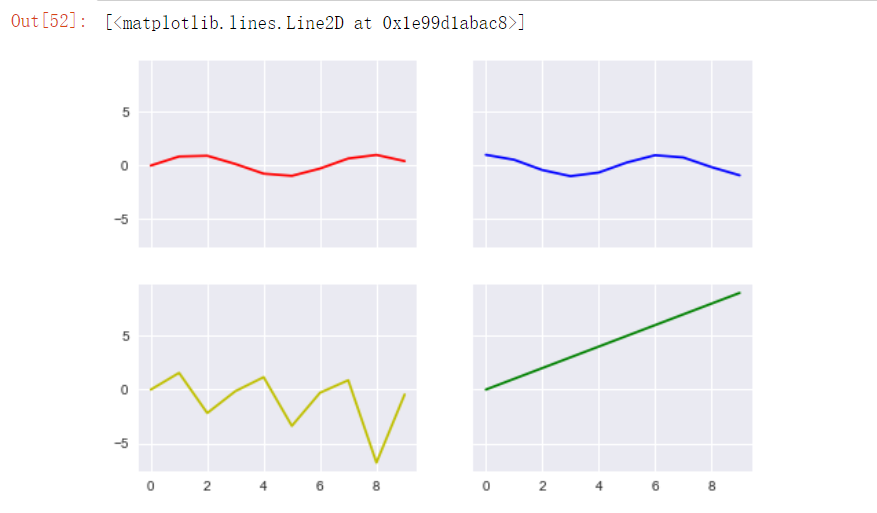### 小结

1. 如果想要在一个图上绘制多根折线，那么直接在plot中传递多个x和y就可以了，或者调用多次plot方法就可以了。
2. 如果想要在一个画板上绘制多个子图（Axes对象），那么可以使用plt.subplot或plt.subplots方法。
3. plt.subplot：plt.subplot(221)，第一个数字代表行，第二个数字代表列，第三个数字代表当前第几个子图。使用了subploth后绘制的所有东西都是在当前子图上，指导出现了新的subplot。
4. plt.subplots：fig,axes = plt.subplots(2,2,sharex=True)，第一个代表行，第二个代表列，这个方法是一次性把所有的子图都绘制了，但是在子图上没有任何的东西，我们需要使用他的返回值来绘制，axes就是子图的对象。

## 6.4 风格设置

matplotlib 图片默认内置了几种风格。我们可以通过 plt.style.available 来查看内置的所有风格: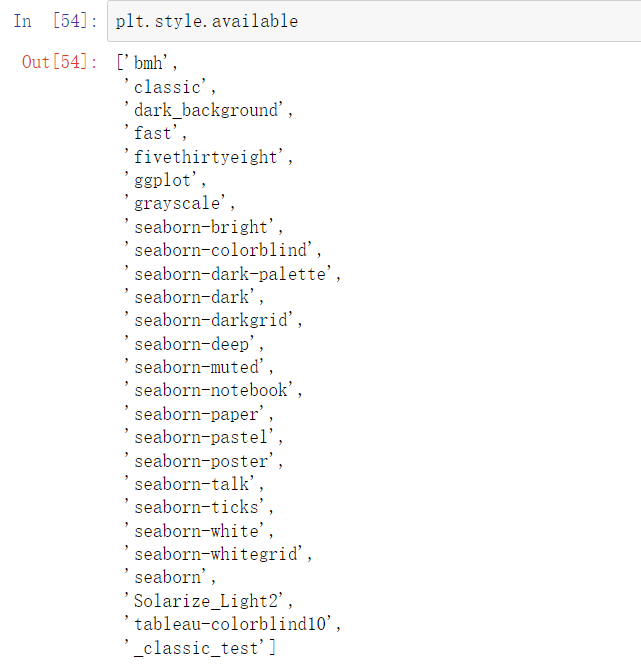``````plt.style.use("dark_background")
figure,axes = plt.subplots(2,2,sharex=True,sharey=True)
axes[0,0].plot(np.sin(np.arange(10)),c='r')
axes[0,1].plot(np.cos(np.arange(10)),c='b')
axes[1,0].plot(np.tan(np.arange(10)),c='y')
axes[1,1].plot(np.arange(10),c='g')
``````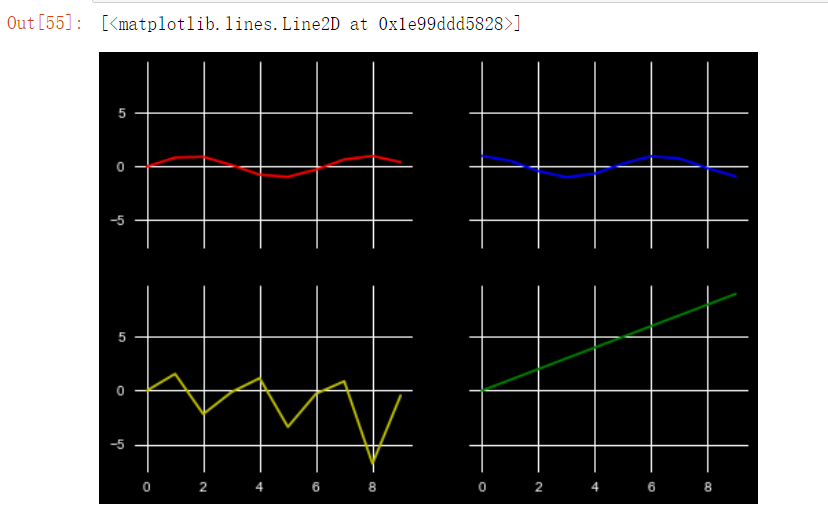# 我将月亮缝入躯体，葬自我于山谷，如果那一天野花疯长，那便是我在讲

[En]

This is the end of the article, I hope this article can be a little help to you, welcome three companies, like, collect something to hide, if there is a problem, feel free to hit me, I have a story, you have wine, good communication, do not break up! Ha ha ha! See you next time!

Original: https://blog.csdn.net/weixin_44327634/article/details/123523501
Author: 冰履踏青云
Title: Matplotlib的基本使用

# 1 查看tensorflow版本

## 1.1 进入对应的环境

``````conda activate tensorflow1140cuda100
``````## 1.2 打开Python

``````python
``````## 1.3 查看tensorflow版本、安装路径

``````import tensorflow as tf
tf.__version__
print(tf.__version__)
tf.__path__
print(tf.__path__)
``````# 2 查看cuda版本、查看cudnn版本

## 2.1 进入对应的环境

``````conda activate tensorflow1140cuda100
``````## 2.2 输入命令，查看cuda版本、cudnn版本

``````conda list | grep cuda

``````# 3 查看GPU可用性

## 3.1 进入对应的环境

``````conda activate tensorflow1140cuda100
``````## 3.2 打开Python

``````python
``````## 3.3 查看GPU可用性

``````import tensorflow as tf
print(tf.test.is_gpu_available())
``````# 4 查看cuda可用性

## 4.1 进入对应的环境

``````conda activate tensorflow1140cuda100
``````## 4.2 打开Python

``````python
``````## 4.3 查看cuda可用性

``````import tensorflow as tf

tf.test.is_built_with_cuda()
print(tf.test.is_built_with_cuda())
``````Original: https://blog.csdn.net/shaqilaixi2/article/details/121777180
Author: C系语言
Title: tensorflow：查看tensorflow版本号、查看cuda版本、查看cudnn版本、查看GPU可用性、查看cuda可用性